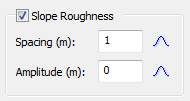Expert Modelling: Hands-on help from Rocscience Engineers. Register Here

# Slope Roughness

The Slope Roughness in the Slope Material Library allows you to account for variability in the local surface angle of segments of the slope. It is important to note the difference in the implementation of Slope Roughness for the Lump Mass method versus the Rigid Body method:

## Lump Mass

The Slope Roughness randomly generates an angle at the point of impact of the point mass. The mean value of the Slope Roughness cannot be changed. It is always equal to the slope segment angle. You can, however, use statistics to account for the variability in the local surface angle of slope segments.

## Rigid Body

The Slope Roughness randomly generates a new slope surface for each rock throw on each applied boundary segment. For Rigid Body analyses, Slope Roughness is defined by Spacing and Amplitude, and statistical distributions can be assigned to each parameter. Note that slope roughness is not applied at vertex locations; vertices remain in their original locations.

Slope Roughness section of Slope Material LibraryThe Spacing is the distance between amplitudes, while the Amplitude is the distance from the base slope. It is recommended to set the mean amplitude equal to zero, so that the roughness is evenly distributed around the base slope (the Amplitude is actually an offset from the mean slope segment).

Slope Roughness with mean Amplitude = 0Slope Roughness with mean Amplitude = 1